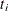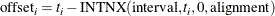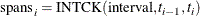# The TIMEID Procedure

### Time ID Diagnostics

For a specified time interval, PROC TIMEID decomposes the raw time ID values in an input data set into the following three quantities, whose values are represented by nonnegative integers at each unique time ID value in the input series:

interval counts

the number of observations that share each time interval in the data set.

offsets

the numerical difference between a time ID value and the aligned value for that time interval. The unit of measure used to express this distance is days for date values and seconds for datetime values. The offset is computed for each time ID value,, by using the following SAS expression:spans

the number of intervals between each time ID value and the previous time ID value. The spans value is equivalent to the number returned by the following SAS expression: